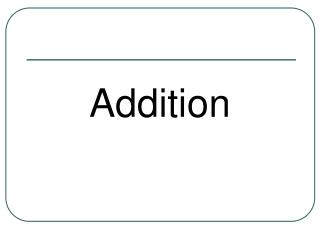Download PresentationAddition - PowerPoint PPT Presentation

Download PresentationAn Image/Link below is provided (as is) to download presentation

Download Policy: Content on the Website is provided to you AS IS for your information and personal use and may not be sold / licensed / shared on other websites without getting consent from its author. While downloading, if for some reason you are not able to download a presentation, the publisher may have deleted the file from their server.

- - - - - - - - - - - - - - - - - - - - - - - - - - - E N D - - - - - - - - - - - - - - - - - - - - - - - - - - -
Presentation Transcript

2. Year R Step 1 • To solve addition using pictures and objects. • No symbols • No sums • Lots of practical activities. • Demonstrate.

3. Picture sums

4. Introduce the language • Add • Addition • Together/Altogether • Equals/Makes • How many? • Count • Plus

5. Year R Step 2 • Count on in 1’s • Board games • Cubes • Fruit/people/toys/milk/cars/doors

6. Year R Expectations • To count to 20 • To count in 2’s,10’s (make it fun) • To know number bonds for 5 • Knowing the language of addition.

7. Year 1 Step 1 • Recording mental addition sums in a number sentence, using correct symbols 1 + 3 = 4 • To count on from a given number in 1’s 7,8,9,10 or 12,13,14,15

8. Year 1 Step 2 • To know addition can be done in any order. 15 + 2 = 2 +15 17 = 15 + 2 Now you try 15 + 6 = 12 +6 = • Count on in 2’s 2,4,6,8,10 and 10’s using splat square

9. Year 1 Step 3 Partitioning • Knowing 12 is 10 + 2 • Knowing 12 + 3 is 10 + 2 + 3 = • Knowing 6 + 4 could be 3 + 3 + 4 = Have a go with these. • 15 + 8 = 11 + 6 = 8 + 5 =

10. Year 1 Expectations • Knowing, and using symbols • Knowing correct vocabulary • Partitioning TU and HTU • Addition doubles • Addition number bonds to 20 • Count on in 1’s 2’s 5’s 10’s • Add on multiples of 10 to 1 and 2 digit numbers.

11. Year 2 Step 1 • To solve addition by partitioning and recombining 12 + 15 10 + 10 2 + 5 20 + 7 = 27

12. Year 2 Step 1 continued • Partitioning within the boundary • 35 + 42 ( Add mentally 30 + 40 then 5 + 2) • Add using partitioning over the boundary • 67+5 • 7 + 5 = 12 • 60 + 10 + 2 = 72

13. Year 2 Step 2 • Empty numbers lines for adding multiples of 10 and then 2 digit numbers. • Demonstrate 42 + 20, then 26 + 14

14. Year 2 Step 3 • Adding using a hundred square. • Counting on • Multiples of 10 • Find number 10 – 1 • Finding 11 10 + 1 14 x =

15. Year 2 Expectations • Know and use symbol • Know all language associated with addition • To add 10, 9, 11 using a 100 square then mentally • To solve addition with partition mentally and through jottings. • Number bonds for 10, 20 and 100